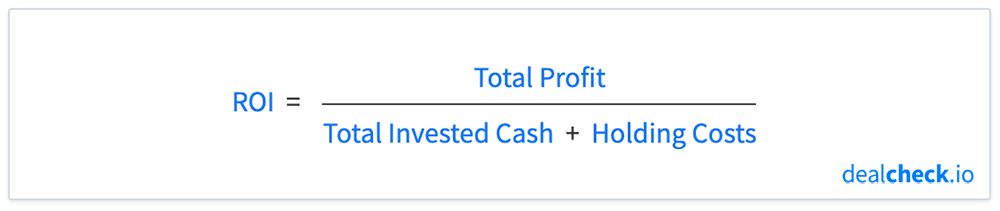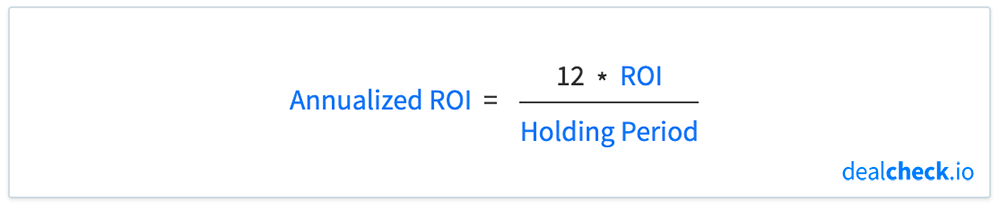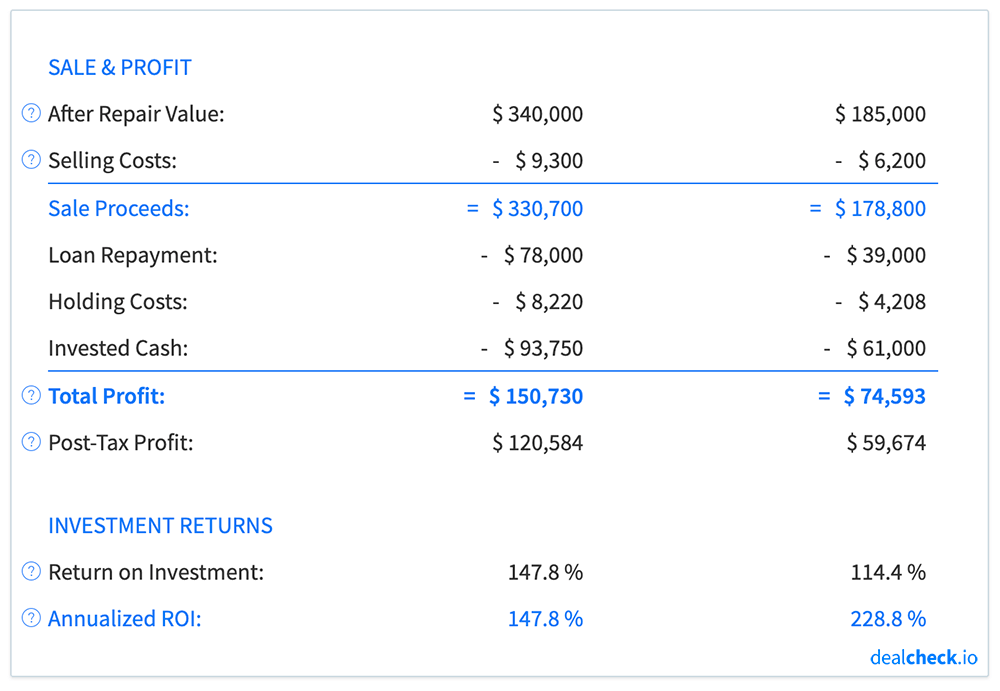Note: This article describes the ROI calculation specifically for flips and rehab projects, and not rental properties, which is calculated differently.

The return on investment, abbreviated as ROI, is a primary measure of investment return used by investors when analyzing and comparing the profitability of house flip projects.

The cumulative ROI metric shows the total return you will receive on your invested capital after completing the rehab work and selling a property for a profit.

The annualized version of the ROI shows a hypothetical yearly rate of return you will receive on your invested capital based on the current flip, and is often a more accurate metric when comparing flips with each other or with other investments.

### The Cumulative Return on Investment Formula

The cumulative return on investment from a fix and flip can be calculated by dividing the total profit by the sum of the total invested cash and holding costs:In the formula above, the total profit is the final net amount you will receive as profit from a flip after selling the property and paying off any outstanding loans.

The total invested cash represents all capital that was originally used to purchase and rehab the property. This usually includes the down payment on the loan (or the purchase price, if not using financing), purchase and closing costs, as well as rehab costs.

The denominator of the formula also includes holding costs, which are all recurring expenses you will incur while rehabbing a property, such as loan payments, dumpster fees, and other overhead costs.

To simplify the above formula, you can simply divide the total profit by all out-of-pocket costs or capital spent during the flip process.

### The Annualized Return on Investment Formula

To calculate the annualized version of the ROI (in other words – the ROI projected to a yearly rate of return), you can multiply the cumulative ROI by 12 and divide it by the holding period of the flip, measured in months:The ROI used in the above formula is the cumulative ROI calculated as described in the previous section.

The holding period is the number of months it will take for you to purchase, rehab and sell the property from start to finish. This is the total number of months you will have the property in your possession throughout the project.

### Return on Investment Example

Let’s assume you found a property for sale for \$100,000, that will need \$50,000 in rehab work and will sell for \$200,000 after it is rehabbed. You also estimate that it will take you 7 months to complete the rehab and sell it, which will be your holding period.

Let’s also assume that your lender is willing to finance 70% of the purchase price of this property and your total holding costs (including loan payments) will be \$10,000 over the course of the rehab period.

First, let’s calculate how much capital you will need to purchase and rehab this property – your total invested cash:

Total Invested Cash = Down Payment + Rehab Costs = \$30,000 + \$50,000 = \$80,000

Next, let’s calculate your total net profit from this project:

Total Profit = Sale Price – Holding Costs – Total Invested Cash = \$200,000 – \$10,000 – \$80,000 = \$110,000

Finally, let’s calculate your cumulative and annualized return on investment:

Cumulative ROI = Total Profit / (Total Invested Cash + Holding Costs) = \$110,000 / (\$80,000 + \$10,000) = 122%

Annualized ROI = 12 * ROI / Holding Period = 12 * 122% / 7 = 210%

### Cumulative ROI vs. Annualized ROI

The main difference between the cumulative and annualized ROI metrics when evaluating flips, is that the cumulative ROI does not take into account how long it will take to rehab and sell a property, while the annualized ROI does take that into account.

Because of this, the annualized ROI is often a better indicator of the profitability of a particular flip, especially when comparing and deciding which projects to take on next.

To illustrate this, let’s compare two hypothetical flip scenarios:

• Flip 1: \$100,000 total investment, 12 month holding period, \$150,000 total profit, 150% cumulative ROI
• Flip 2: \$65,000 total investment, 6 month holding period, \$75,000 total profit, 115% cumulative ROI

Looking at the two examples above, at first glance you may believe that Flip 1 is a better investment, since it will net a larger total profit and a higher cumulative return on your investment.

But let’s calculate the annualized ROI for each of these projects:

• Flip 1: 150% annualized ROI
• Flip 2: 230% annualized ROI

As you can see, the annualized ROI is much higher for the second flip. This is because its projected holding period is much shorter, even though the total profit and cumulative ROI are less.

Choosing projects similar to Flip 2 above will allow you to purchase, rehab and sell more properties for a larger total profit over the course of the year, instead of tying up your capital in longer projects similar to Flip 1.Of course, it’s important to consider all factors when choosing properties to flip, but you may want to pay special attention to the annualized ROI metric, instead of focusing exclusively on the total profit and cumulative ROI.

### Calculate ROI and Dozens of Other Metrics in Seconds

The DealCheck property analysis app makes it easy to calculate the return on investment, along with dozens of other property analysis metrics for house flips and rehab projects in seconds.